## Tree Data Structure-

Tree data structure may be defined as-

 Tree is a non-linear data structure which organizes data in a hierarchical structure and this is a recursive definition.ORA tree is a connected graph without any circuits.ORIf in a graph, there is one and only one path between every pair of vertices, then graph is called as a tree.

## Example-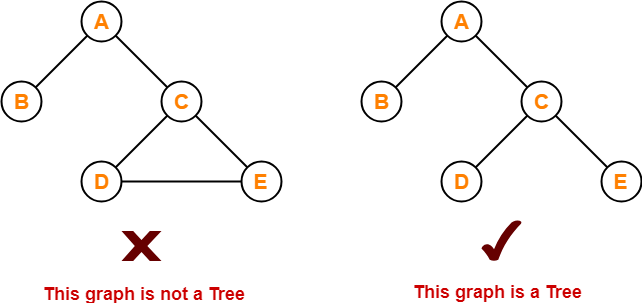## Properties-

The important properties of tree data structure are-

• There is one and only one path between every pair of vertices in a tree.
• A tree with n vertices has exactly (n-1) edges.
• A graph is a tree if and only if it is minimally connected.
• Any connected graph with n vertices and (n-1) edges is a tree.

To gain better understanding about Tree Data Structure,

Watch this Video Lecture

## Tree Terminology-

The important terms related to tree data structure are-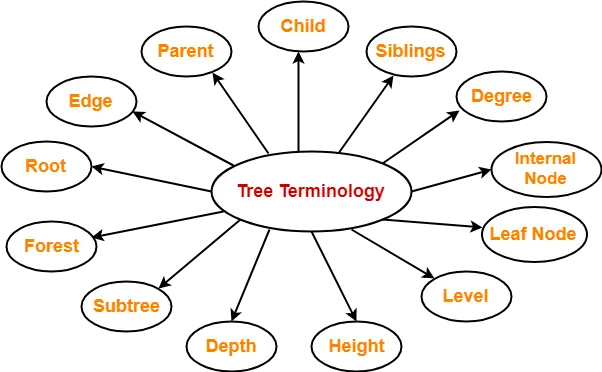## 1. Root-

• The first node from where the tree originates is called as a root node.
• In any tree, there must be only one root node.
• We can never have multiple root nodes in a tree data structure.

### Example-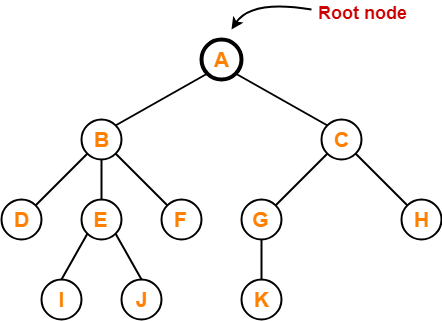Here, node A is the only root node.

## 2. Edge-

• The connecting link between any two nodes is called as an edge.
• In a tree with n number of nodes, there are exactly (n-1) number of edges.

### Example-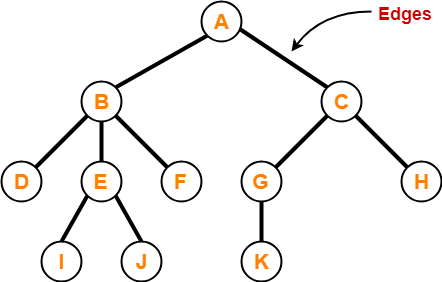## 3. Parent-

• The node which has a branch from it to any other node is called as a parent node.
• In other words, the node which has one or more children is called as a parent node.
• In a tree, a parent node can have any number of child nodes.

### Example-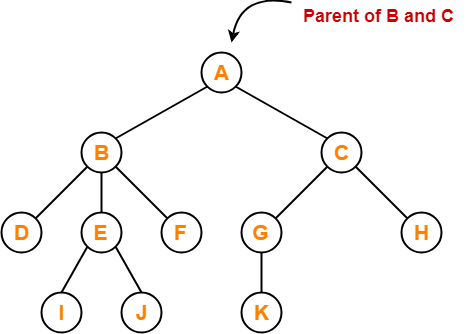Here,

• Node A is the parent of nodes B and C
• Node B is the parent of nodes D, E and F
• Node C is the parent of nodes G and H
• Node E is the parent of nodes I and J
• Node G is the parent of node K

## 4. Child-

• The node which is a descendant of some node is called as a child node.
• All the nodes except root node are child nodes.

### Example-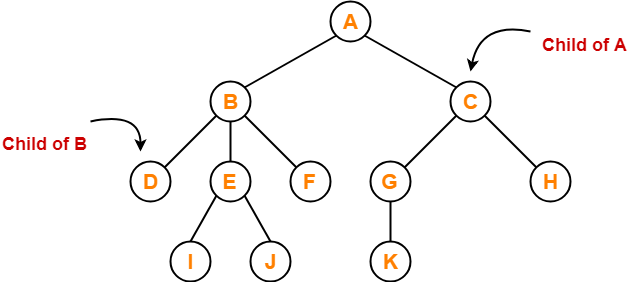Here,

• Nodes B and C are the children of node A
• Nodes D, E and F are the children of node B
• Nodes G and H are the children of node C
• Nodes I and J are the children of node E
• Node K is the child of node G

## 5. Siblings-

• Nodes which belong to the same parent are called as siblings.
• In other words, nodes with the same parent are sibling nodes.

### Example-Here,

• Nodes B and C are siblings
• Nodes D, E and F are siblings
• Nodes G and H are siblings
• Nodes I and J are siblings

## 6. Degree-

• Degree of a node is the total number of children of that node.
• Degree of a tree is the highest degree of a node among all the nodes in the tree.

### Example-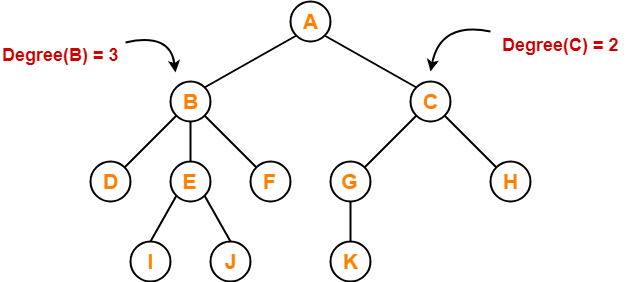Here,

• Degree of node A = 2
• Degree of node B = 3
• Degree of node C = 2
• Degree of node D = 0
• Degree of node E = 2
• Degree of node F = 0
• Degree of node G = 1
• Degree of node H = 0
• Degree of node I = 0
• Degree of node J = 0
• Degree of node K = 0

## 7. Internal Node-

• The node which has at least one child is called as an internal node.
• Internal nodes are also called as non-terminal nodes.
• Every non-leaf node is an internal node.

### Example-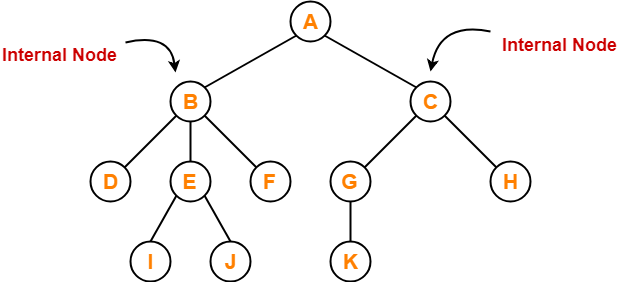Here, nodes A, B, C, E and G are internal nodes.

## 8. Leaf Node-

• The node which does not have any child is called as a leaf node.
• Leaf nodes are also called as external nodes or terminal nodes.

### Example-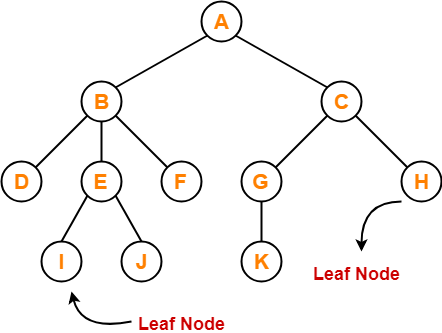Here, nodes D, I, J, F, K and H are leaf nodes.

## 9. Level-

• In a tree, each step from top to bottom is called as level of a tree.
• The level count starts with 0 and increments by 1 at each level or step.

### Example-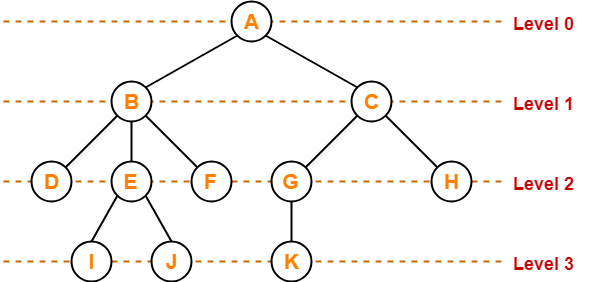## 10. Height-

• Total number of edges that lies on the longest path from any leaf node to a particular node is called as height of that node.
• Height of a tree is the height of root node.
• Height of all leaf nodes = 0

### Example-Here,

• Height of node A = 3
• Height of node B = 2
• Height of node C = 2
• Height of node D = 0
• Height of node E = 1
• Height of node F = 0
• Height of node G = 1
• Height of node H = 0
• Height of node I = 0
• Height of node J = 0
• Height of node K = 0

## 11. Depth-

• Total number of edges from root node to a particular node is called as depth of that node.
• Depth of a tree is the total number of edges from root node to a leaf node in the longest path.
• Depth of the root node = 0
• The terms “level” and “depth” are used interchangeably.

### Example-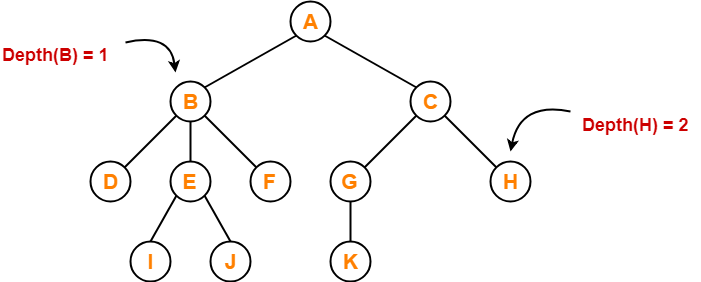Here,

• Depth of node A = 0
• Depth of node B = 1
• Depth of node C = 1
• Depth of node D = 2
• Depth of node E = 2
• Depth of node F = 2
• Depth of node G = 2
• Depth of node H = 2
• Depth of node I = 3
• Depth of node J = 3
• Depth of node K = 3

## 12. Subtree-

• In a tree, each child from a node forms a subtree recursively.
• Every child node forms a subtree on its parent node.

### Example-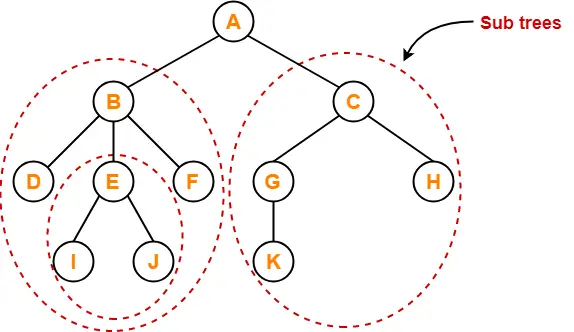## 13. Forest-

A forest is a set of disjoint trees.

### Example-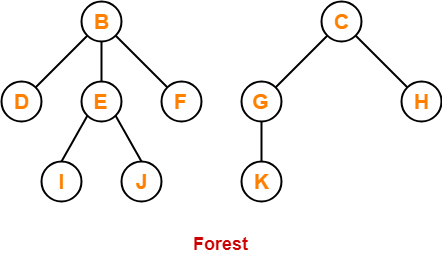To gain better understanding about Tree Terminology,

Watch this Video LectureNext Article- Binary Tree | Types of Binary Trees

Get more notes and other study material of Data Structures.

Watch video lectures by visiting our YouTube channel LearnVidFun.